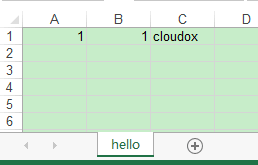# 零基础使用Python读写处理Excel表格的方法

更新时间：2019年05月02日 10:01:10   作者：Cloudox_我要评论

Python自己是不支持直接操作Excel的，但是Python强大之处就在于有大量好用的第三方库，这里我们选用读Excel的xlrd库和写Excel的xlwt库来操作。

* 下载xlrd
* 下载xlwt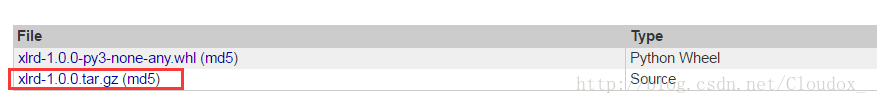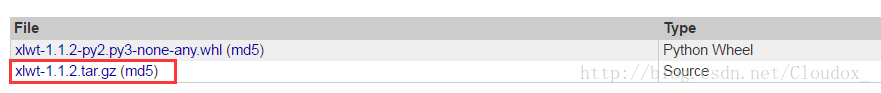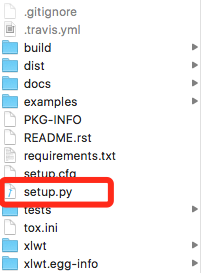\$ cd xlwt-1.1.2
\$ sudo python setup.py install

xlrd 也是同样的安装方式。

```# -*- coding: utf-8 -*-
import xdrlib ,sys
import xlrd

#打开excel文件
def open_excel(file= 'test.xlsx'):
try:
data = xlrd.open_workbook(file)
return data
except Exception,e:
print str(e)

#根据名称获取Excel表格中的数据  参数:file：Excel文件路径   colnameindex：表头列名所在行的索引 ，by_name：Sheet1名称
def excel_table_byname(file= 'test.xlsx', colnameindex=0, by_name=u'Sheet1'):
data = open_excel(file) #打开excel文件
table = data.sheet_by_name(by_name) #根据sheet名字来获取excel中的sheet
nrows = table.nrows #行数
colnames = table.row_values(colnameindex) #某一行数据
list =[] #装读取结果的序列
for rownum in range(0, nrows): #遍历每一行的内容
row = table.row_values(rownum) #根据行号获取行
if row: #如果行存在
app = [] #一行的内容
for i in range(len(colnames)): #一列列地读取行的内容
app.append(row[i])
list.append(app) #装载数据
return list

#主函数
def main():
tables = excel_table_byname()
for row in tables:
print row

if __name__=="__main__":
main()
```

Excel中内容如下：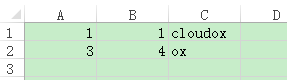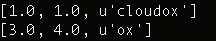```# -*- coding: utf-8 -*-
import xlwt

def testXlwt(file = 'new.xls'):
book = xlwt.Workbook() #创建一个Excel
sheet1.write(0,0,'cloudox') #往sheet里第一行第一列写一个数据
sheet1.write(1,0,'ox') #往sheet里第二行第一列写一个数据
book.save(file) #创建保存文件

#主函数
def main():
testXlwt()

if __name__=="__main__":
main()
```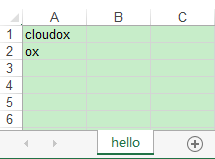Library to create spreadsheet files compatible with MS Excel 97/2000/XP/2003 XLS files, on any platform, with Python 2.6, 2.6, 3.3+

```# -*- coding: utf-8 -*-
import xdrlib ,sys
import xlrd
import xlwt

#打开excel文件
def open_excel(file= 'test.xlsx'):
try:
data = xlrd.open_workbook(file)
return data
except Exception,e:
print str(e)

#根据索引获取Excel表格中的数据  参数:file：Excel文件路径   colnameindex：表头列名所在行的索引 ，by_index：表的索引
def excel_table_byindex(file= 'test.xlsx',colnameindex=0,by_index=0):
data = open_excel(file) #打开excel文件
table = data.sheets()[by_index] #根据sheet序号来获取excel中的sheet
nrows = table.nrows #行数
ncols = table.ncols #列数
colnames = table.row_values(colnameindex) #某一行数据
list =[] #装读取结果的序列
for rownum in range(0,nrows): #遍历每一行的内容

row = table.row_values(rownum) #根据行号获取行
if row: #如果行存在
app = [] #一行的内容
for i in range(len(colnames)): #一列列地读取行的内容
app.append(row[i])
if app == app : #如果这一行的第一个和第二个数据相同才将其装载到最终的list中
list.append(app)
testXlwt('new.xls', list) #调用写函数，讲list内容写到一个新文件中
return list

#将list中的内容写入一个新的file文件
def testXlwt(file = 'new.xls', list = []):
book = xlwt.Workbook() #创建一个Excel
i = 0 #行序号
for app in list : #遍历list每一行
j = 0 #列序号
for x in app : #遍历该行中的每个内容（也就是每一列的）
sheet1.write(i, j, x) #在新sheet中的第i行第j列写入读取到的x值
j = j+1 #列号递增
i = i+1 #行号递增
# sheet1.write(0,0,'cloudox') #往sheet里第一行第一列写一个数据
# sheet1.write(1,0,'ox') #往sheet里第二行第一列写一个数据
book.save(file) #创建保存文件

#主函数
def main():
tables = excel_table_byindex()
for row in tables:
print row

if __name__=="__main__":
main()
```